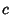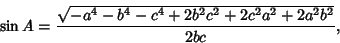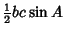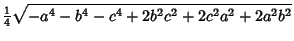## Heron's Formula

Gives the Area of a Triangle in terms of the lengths of the sides,, andand the Semiperimeter(1)

Heron's formula then states(2)

Expressing the side lengths,, andin terms of the radii,, and' of the mutually tangent circles centered on the Triangle vertices (which define the Soddy Circles),(3)(4)(5)

gives the particularly pretty form(6)

The proof of this fact was discovered by Heron(ca. 100 BC-100 AD), although it was already known to Archimedesprior to 212 BC (Kline 1972). Heron's proof (Dunham 1990) is ingenious but extremely convoluted, bringing together a sequence of apparently unrelated geometric identities and relying on the properties of Cyclic Quadrilaterals and Right Triangles.

Heron's proof can be found in Proposition 1.8 of his work Metrica. This manuscript had been lost for centuries until a fragment was discovered in 1894 and a complete copy in 1896 (Dunham 1990, p. 118). More recently, writings of the Arab scholar Abu'l Raihan Muhammed al-Biruni have credited the formula to Heron's predecessor Archimedes(Dunham 1990, p. 127).

A much more accessible algebraic proof proceeds from the Law of Cosines,(7)

Then(8)

giving(9)(10)(11)(12)

(Coxeter 1969). Heron's formula contains the Pythagorean Theorem.

See also Brahmagupta's Formula, Bretschneider's Formula, Heronian Tetrahedron, Heronian Triangle, Soddy Circles, SSS Theorem, Triangle

References

Coxeter, H. S. M. Introduction to Geometry, 2nd ed. New York: Wiley, p. 12, 1969.

Dunham, W. Heron's Formula for Triangular Area.'' Ch. 5 in Journey Through Genius: The Great Theorems of Mathematics. New York: Wiley, pp. 113-132, 1990.

Kline, M. Mathematical Thought from Ancient to Modern Times. New York: Oxford University Press, 1972.

Pappas, T. Heron's Theorem.'' The Joy of Mathematics. San Carlos, CA: Wide World Publ./Tetra, p. 62, 1989.